Chapter 3 Class 12 Matrices
Concept wise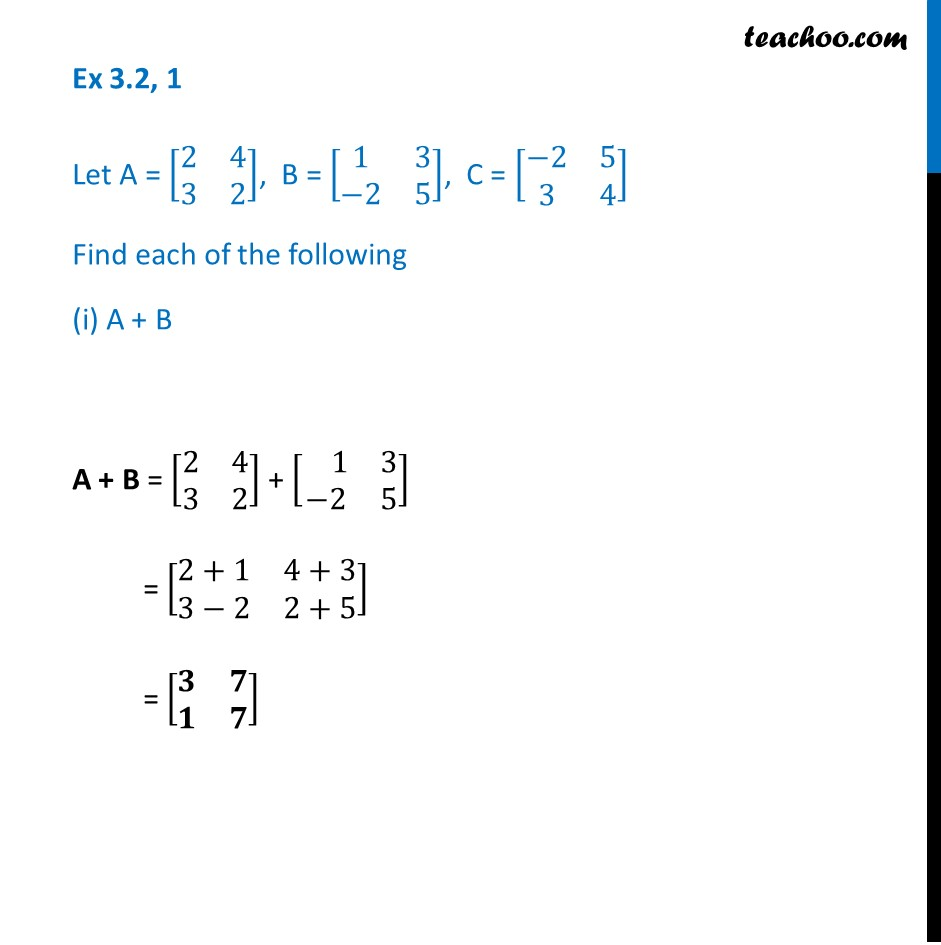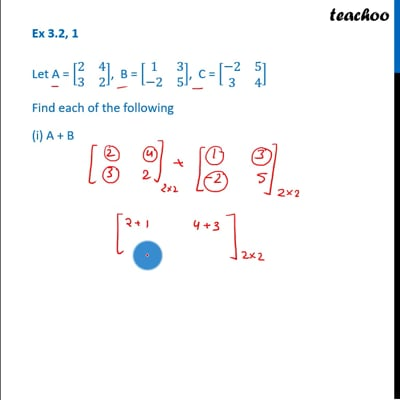This video is only available for Teachoo black users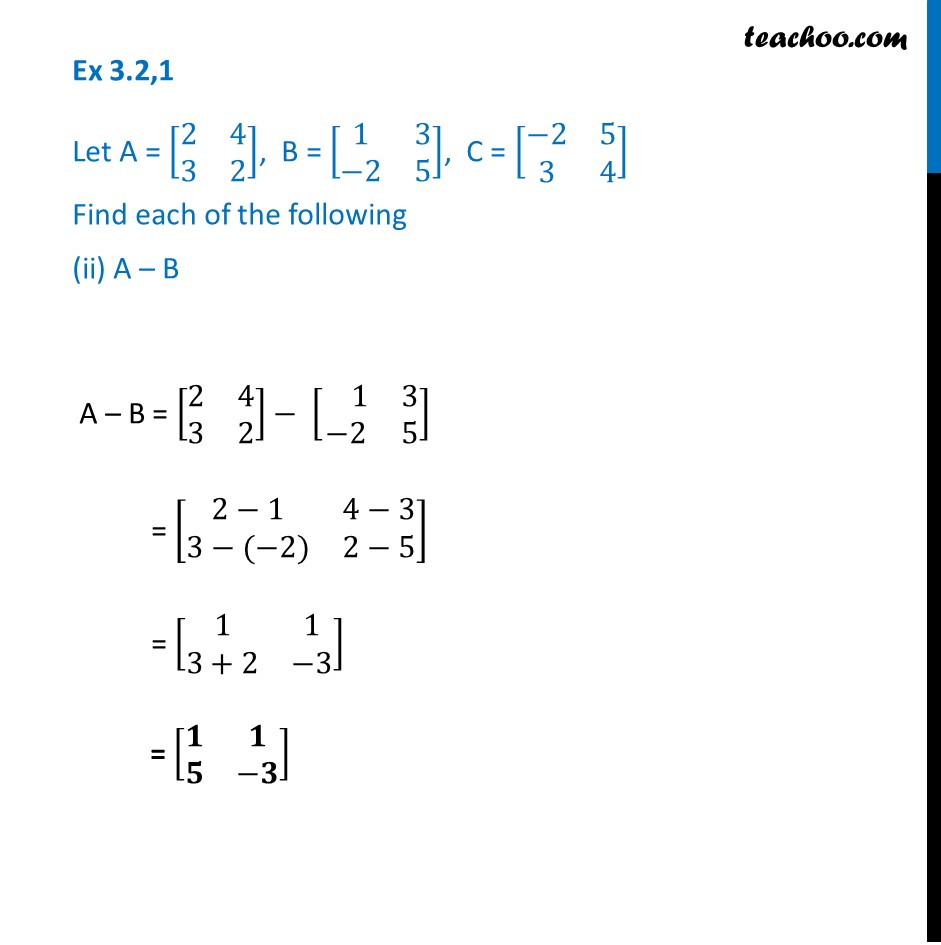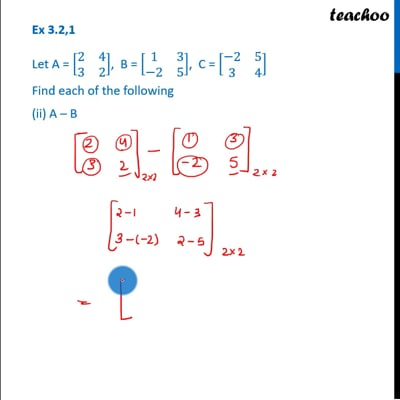This video is only available for Teachoo black users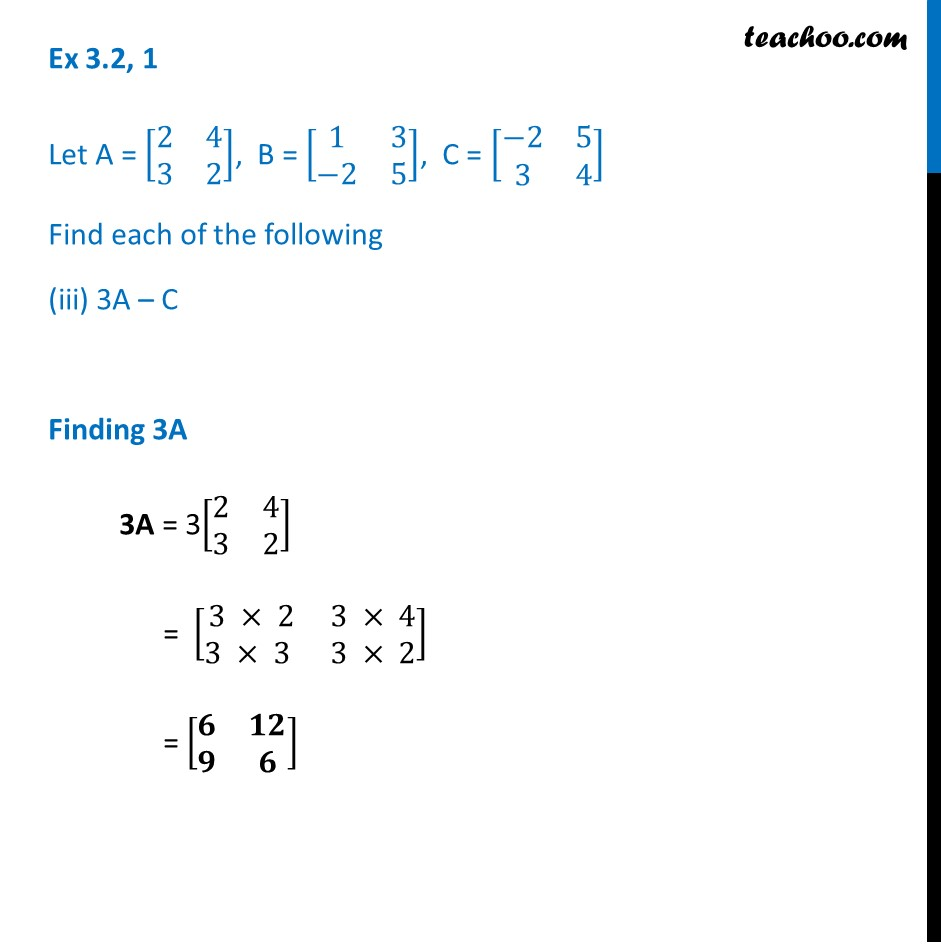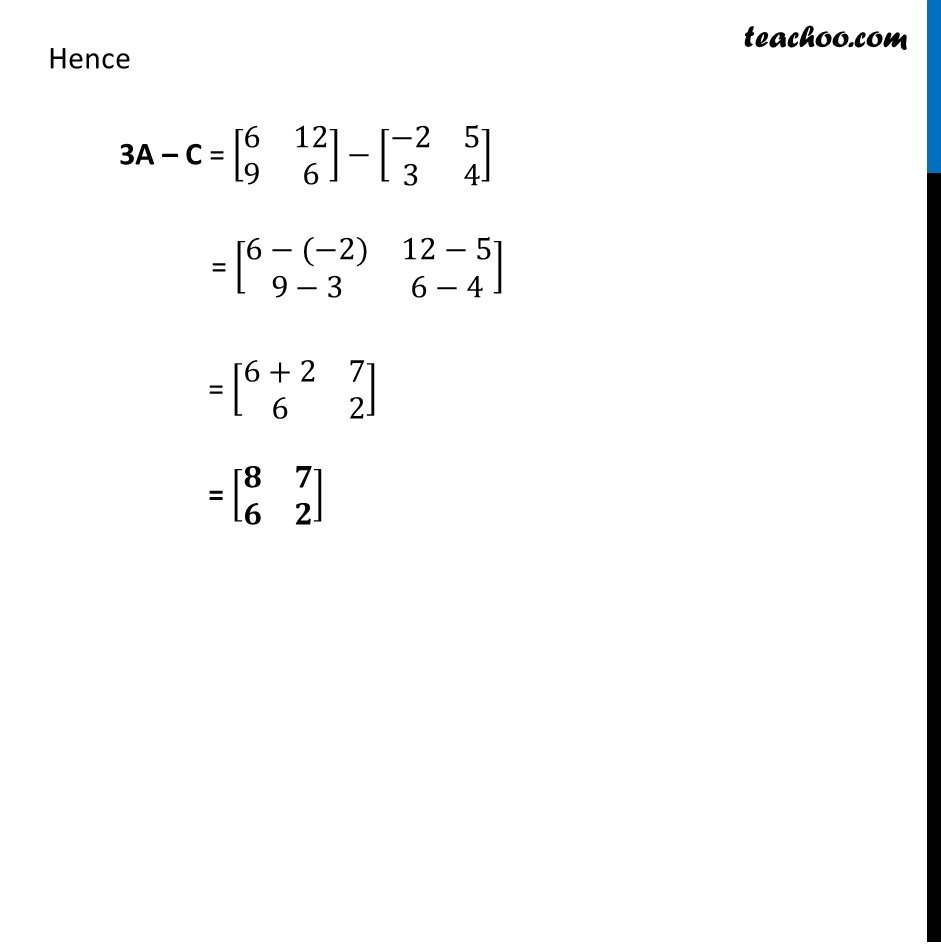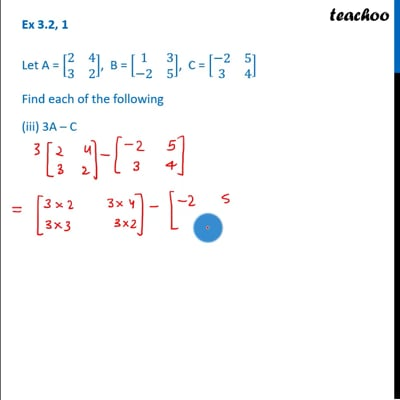This video is only available for Teachoo black users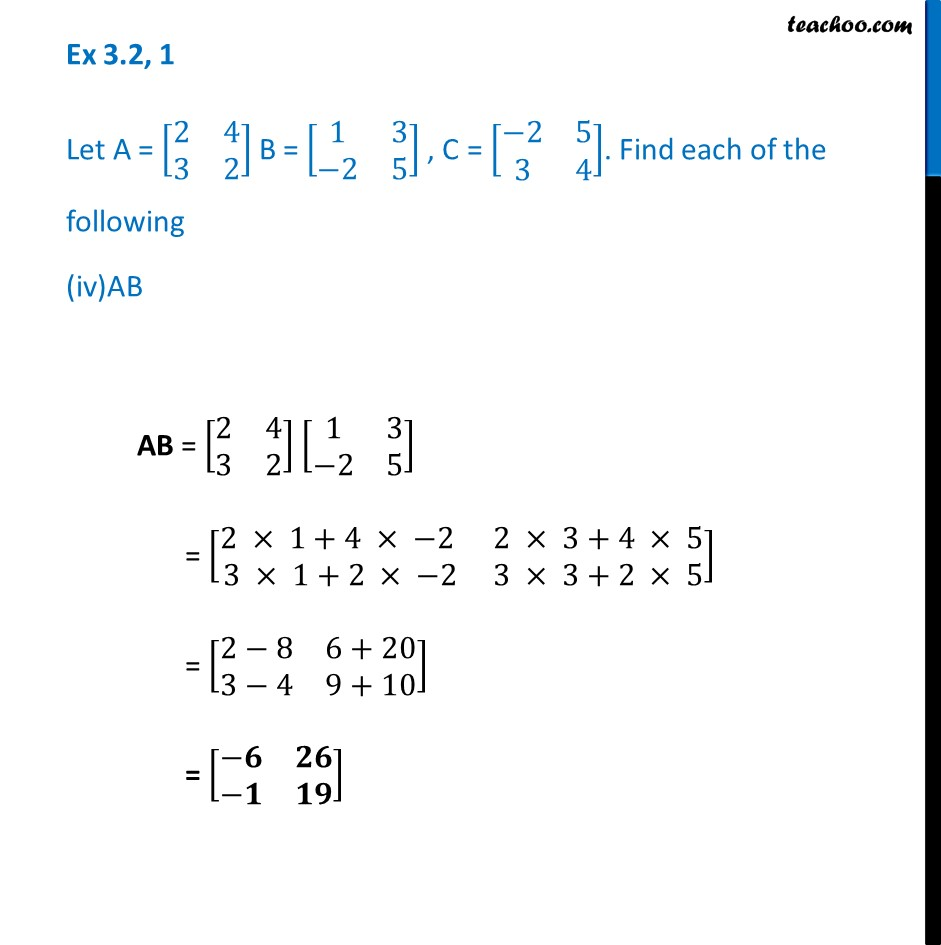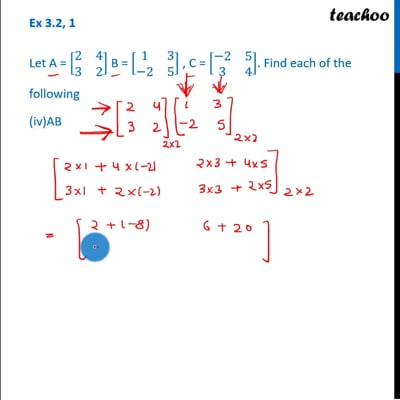This video is only available for Teachoo black users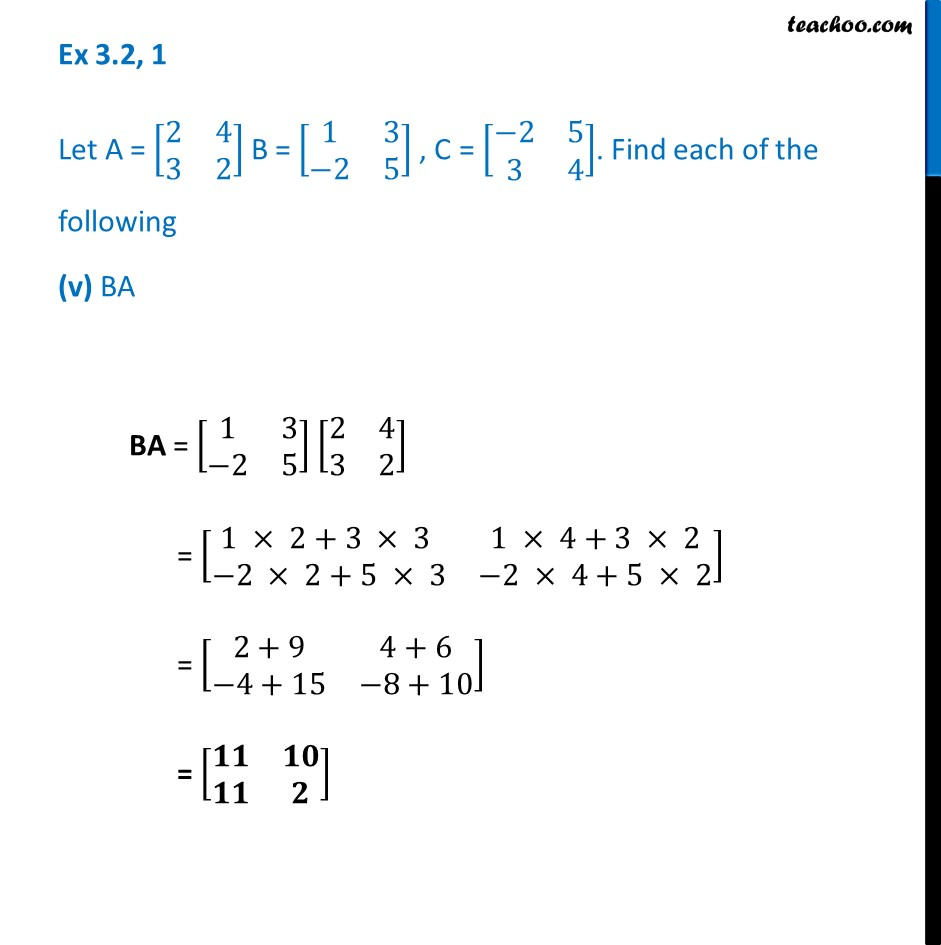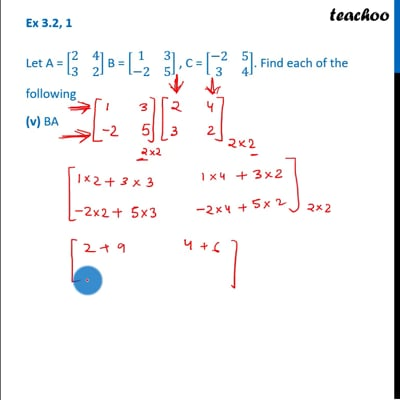This video is only available for Teachoo black users

Learn in your speed, with individual attention - Teachoo Maths 1-on-1 Class

### Transcript

Ex 3.2, 1 Let A = [■8(2&4@3&2)], B = [■8(1&3@−2&5)], C = [■8(−2&5@3&4)] Find each of the following (i) A + B A + B = [■8(2&4@3&2)] + [■8( 1&3@−2&5)] = [■8(2+1&4+3@3−2&2+5)] = [■8(𝟑&𝟕@𝟏&𝟕)] Ex 3.2,1 Let A = [■8(2&4@3&2)], B = [■8(1&3@−2&5)], C = [■8(−2&5@3&4)] Find each of the following (ii) A – B A – B = [■8(2&4@3&2)]− [■8( 1&3@−2&5)] = [■8(2−1&4−3@3−(−2)&2−5)] = [■8(1&1@3+2&−3)] = [■8(𝟏&𝟏@𝟓&−𝟑)] Ex 3.2, 1 Let A = [■8(2&4@3&2)], B = [■8(1&3@−2&5)], C = [■8(−2&5@3&4)] Find each of the following 3A – C Finding 3A 3A = 3[■8(2&4@3&2)] = [■8(3 × 2&3 × 4@3 × 3 &3 × 2)] = [■8(𝟔&𝟏𝟐@𝟗&𝟔)] Hence 3A – C = [■8(6&12@9&6)] ⤶7− [■8(−2&5@3&4)] = [■8(6−(−2)&12−5@9−3&6−4)] = [■8(6+2&7@6&2)] = [■8(𝟖&𝟕@𝟔&𝟐)] Ex 3.2, 1 Let A = [■8(2&4@3&2)] B = [■8(1&3@−2&5)] , C = [■8(−2&5@3&4)]. Find each of the following (iv)AB AB = [■8(2&4@3&2)] [■8(1&3@−2&5)] = [■8(2 × 1+4 × −2 &2 × 3+4 × 5@3 × 1+2 × −2&3 × 3+2 × 5)] = [■8(2−8&6+20@3−4&9+10)] = [■8(−𝟔&𝟐𝟔@−𝟏&𝟏𝟗)] Ex 3.2, 1 Let A = [■8(2&4@3&2)] B = [■8(1&3@−2&5)] , C = [■8(−2&5@3&4)]. Find each of the following (v) BA BA = [■8(1&3@−2&5)] [■8(2&4@3&2)] = [■8(1 × 2+3 × 3 &1 × 4+3 × 2@−2 × 2+5 × 3&−2 × 4+5 × 2)] = [■8(2+9&4+6@−4+15&−8+10)] = [■8(𝟏𝟏&𝟏𝟎@𝟏𝟏&𝟐)]